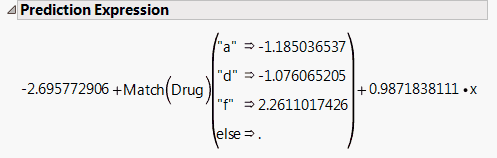Publication date: 11/29/2021

## Show Prediction Expression

The Show Prediction Expression option shows the equation used to predict the response. Figure 3.20 shows an example for the Drug.jmp sample data table. This expression is given as a typical JMP formula. For example, to predict the response for someone on Drug a with x = 10, you would calculate, with some rounding: –2.696 - 1.185 + 0.987(10) = 5.99.

Tip: To specify the number of digits in the prediction formula, go to File > Preferences > Tables and change the Default Field Width value.

Figure 3.20 Prediction ExpressionTo obtain the preceding report, follow these steps:

#### Example of a Prediction Expression

1. Select Help > Sample Data Library and open Drug.jmp.

2. Select Analyze > Fit Model.

3. Select y and click Y.

4. Select Drug and x, and then click Add.

5. Click Run.

6. Click the Response y red triangle and select Estimates > Show Prediction Expression. The report in Figure 3.20 appears.